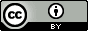## Saturday, April 16, 2016

### Computing pKa values for molecules with several ionizable groups

We're working on pKa prediction using semiempirical methods and need to compute pKa values  for molecules with several ionizable groups. Here are my current thoughts so far.

Background: one ionizable group
If there is only one tritrateable site
$$\mathrm{BH \rightleftharpoons B + H^+} \ \ \ K=\mathrm{\frac{[B][H^+]}{[BH]}}$$
then the fraction of $\mathrm{BH}$ molecules $f_{\mathrm{BH}}$ is $$\begin{split} f_{\mathrm{BH}} & =\mathrm{\frac{[BH]}{[B]+[BH]} } \\ & = \mathrm{\frac{[B]}{[B]}\frac{[BH]/[B]}{1+[BH]/[B]} } \\ & = \mathrm{\frac{[H^+]/K}{1+[H^+]/K} } \\ & = \mathrm{\frac{10^{p\textit{K}-pH}}{1+10^{p\textit{K}-pH}} } \end{split}$$ where $$\mathrm{pH = -log[H^+] \implies [H^+] = 10^{-pH}}$$
and similarly for $K$.

From this we can see that the pK value is the pH value for which $f_{\mathrm{BH}}$ = 1/2. So you compute the pK value from the standard free energy difference
$$\text{p}K =\left( \Delta G^\circ(\mathrm{B})+\Delta G^\circ(\mathrm{H^+})- \Delta G^\circ(\mathrm{BH})\right)/RT\ln(10)$$
and you're done.

Two ionizable groups
For a molecule with two titrateable groups ($\mathrm{HB_\alpha B_\beta H}$) and the following equilibrium constants $$\mathrm{HBBH \rightleftharpoons BBH + H^+} \ \ K_{\alpha1}$$ $$\mathrm{HBBH \rightleftharpoons HBB + H^+} \ \ K_{\beta1}$$ $$\mathrm{HBB \rightleftharpoons BB + H^+} \ \ K_{\alpha0}$$ $$\mathrm{BBH \rightleftharpoons BB + H^+} \ \ K_{\beta0}$$ The probability of, for example, $\mathrm{BBH}$ is $$f_{\mathrm{BBH}} =\mathrm{\frac{[BBH]}{[BB]+[BBH]+[HBB]+[HBBH]}= \frac{[BBH]}{\textit{P}}}$$ $f_{\mathrm{BBH}}$ can be rewritten in terms of pK values $$f_{\mathrm{BBH}} = \mathrm{\frac{[BBH]/[BB]}{\textit{P}/[BB]} = \frac{10^{p\textit{K}_{\beta0}-pH}}{\textit{P}/[BB]}}$$ where $$\mathrm{ \textit{P}/[BB] = 1+10^{p\textit{K}_{\alpha0}-pH}+10^{p\textit{K}_{\beta0}-pH}+ 10^{p\textit{K}_{\alpha0}+p\textit{K}_{\beta1}-2pH}}$$ Similarly, $$f_{\mathrm{HBB}} = \mathrm{\frac{10^{p\textit{K}_{\alpha0}-pH}}{\textit{P}/[BB]}}$$ and $$f_{\mathrm{HBBH}} = \mathrm{\frac{10^{p\textit{K}_{\alpha0}+p\textit{K}_{\beta1}-2pH}}{\textit{P}/[BB]}}$$  The apparent pK value of the $\alpha$ group ($\mathrm{p}K_{\alpha}$) is the pH at which its protonation probability $$f_\alpha =f_{\mathrm{HBB}} + f_{\mathrm{HBBH}}$$ is 1/2 and similarly for the $\beta$ group.  So compute the microscopic pK values (Eq 4-7), then $f_\alpha$ and $f_\beta$ as a function of pH, and then $\mathrm{p}K_{\alpha}$ and $\mathrm{p}K_{\beta}$

If one of the groups (say $\alpha$) titrates at a significantly lower pH than the other ($\mathrm{p}K_{\alpha1} << \mathrm{p}K_{\beta1}$) then $\mathrm{p}K_{\alpha}=\mathrm{p}K_{\alpha1}$ and $\mathrm{p}K_{\beta}=\mathrm{p}K_{\beta0}$ and it is not necessary to compute the free energy of $\mathrm{HBB}$, but it can be hard to determine this in advance.  Similarly, if there is no significant interaction between the sites then $\mathrm{p}K_{\alpha}=\mathrm{p}K_{\alpha1}=\mathrm{p}K_{\alpha0}$ and $\mathrm{p}K_{\beta}=\mathrm{p}K_{\beta1}=\mathrm{p}K_{\beta0}$ and one can skip one of the protonation states.

For $N$ ionizable groups one has to determine $2^N$ microscopic pKa values, which quickly gets out of hand if one has to do a conformational search for each protonation state and the molecule is large.

Related post
Generating protonation states and conformations